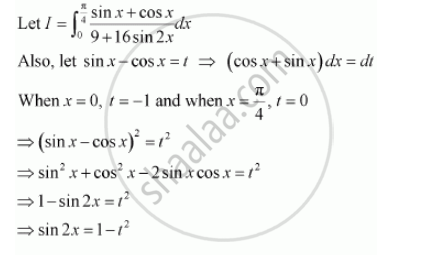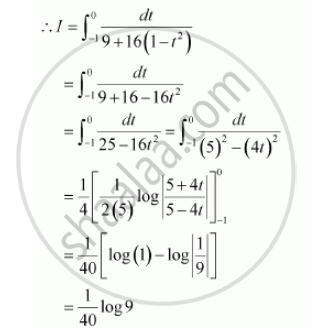Share

# Evaluate the Definite Integrals Int_0^(Pi/4) (Sin X + Cos X)/(9+16sin 2x) Dx - CBSE (Science) Class 12 - Mathematics

ConceptDefinite Integral as the Limit of a Sum

#### Question

Evaluate the definite integrals int_0^(pi/4) (sin x +  cos x)/(9+16sin 2x) dx

#### SolutionIs there an error in this question or solution?

#### Video TutorialsVIEW ALL 

Solution Evaluate the Definite Integrals Int_0^(Pi/4) (Sin X + Cos X)/(9+16sin 2x) Dx Concept: Definite Integral as the Limit of a Sum.
S